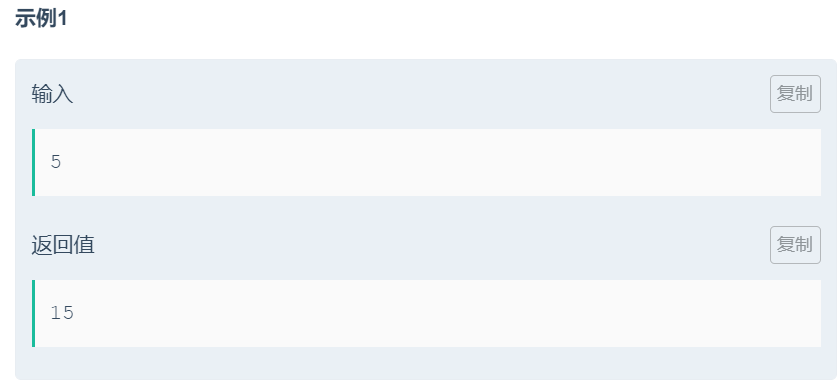## 【刷题】求1+2+3+...+n，要求不能使用乘除法、for、while、if、else、switch、case等关键字及条件判断语句（A?B:C）。

2021/2/13 18:18:58 文章标签: 测试文章如有侵权请发送至邮箱809451989@qq.com投诉后文章立即删除

## 题目## 解答：

``````class Solution
{
public:
// 内部类
class Sum
{
public:
// 构造函数
Sum()
{
// 内部类直接访问外部类的私有成员
_sum+=_num;
_num++;
}
};

int Sum_Solution(int n)
{
// 对两个静态成员变量进行一个重置操作
// 在测试用例的时候，两个变量没有重置，就会累加
_sum=0;
_num=1;
// 调用n次Sum类的构造函数，创建Sum类对象数组
// 此编译器下支持n为变量
Sum SumArray[n];
return _sum;
}
private:
// 声明
static int _sum;
static int _num;
};

// 类外初始化
int Solution::_sum=0;
int Solution::_num=1;
``````

暂无相关的数据...